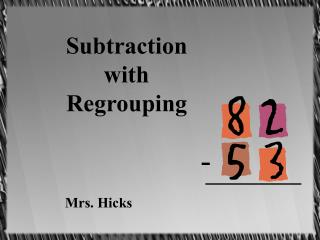DownloadDownload PresentationSubtraction with Regrouping

# Subtraction with Regrouping

Télécharger la présentation## Subtraction with Regrouping

- - - - - - - - - - - - - - - - - - - - - - - - - - - E N D - - - - - - - - - - - - - - - - - - - - - - - - - - -
##### Presentation Transcript

1. Subtraction with Regrouping Mrs. Hicks -

2. 1. Look at the ones. Ask: “Can we take 3 away from 2?” 8 2 -5 3 If we can’t, then we have to regroup.

3. 2. Regroup as needed. 7 8 2 1st: Go next door to the tens and take one away. 1 2nd: Add the ten to the ones. -5 3

4. 3. Subtract the ones. 7 What is 12 – 3? 8 2 1 -5 3 9

5. 4. Subtract the tens. 7 What is 7 – 5? 8 2 1 -5 3 2 9

6. Now let’s review the steps… • Look at the ones. Ask: Can we subtract? • Regroup as needed: 1. Go to the tens and take one away. 2. Add the ten to the ones. • Subtract the ones. • Subtract the tens.

7. We can check our subtraction with addition! 7 8 2 2 9 1 +5 3 -5 3 2 9 1st: Move the answer ’29’ to the top of the addition problem. 2nd: Move the subtracted number ’53’ across to complete the addition problem.

8. Now we’re ready to add… 1st:Add the ones: 9 + 3 2nd:Regroup the ten. 1 7 8 2 2 9 1 +5 3 -5 3 8 2 2 9 3rd: Add the tens – Don’t forget to add the regrouped one!

9. Let’s see if we are right… Yes!! They match! We are right! 1 7 8 2 2 9 1 +5 3 -5 3 8 2 2 9 Does the answer of the addition problem ’82’ match the top number of the subtraction problem?

10. Great Job! Now it’s your turn to prove you’re the math wizard!